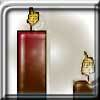You may also likeBurning Down

One night two candles were lit. Can you work out how long each candle was originally?Percentage Unchanged

If the base of a rectangle is increased by 10% and the area is unchanged, by what percentage is the width decreased by ?Digit Sum

What is the sum of all the digits in all the integers from one to one million?

The Power of the Sum

Age 14 to 16 ShortChallenge Level

Answer: $n=7$

Using pairs of equal numbers
\begin{align}&2^6+2^5+2^4+2^4\\ =&2^6 + 2^5 + 2^4\times2\\ =&2^6 + 2^5 + 2^{4+1}\\ =&2^6 + 2^5 + 2^5\\ =&2^6 + 2^5\times2\\ =&2^6 + 2^6\\ =&2^7\end{align}

Finding the value of the powers of 2
$2^2=4$                 $2^5=32$
$2^3=8$                 $2^6=64$
$2^4=16$               $2^7=128$

So $2^6+2^5+2^4+2^4=64+32+16+16=128=2^7$

Factorising and using index laws
Notice that all of the numbers in the sum are multiples of $2^4$, since $2^6=2^2\times2^4,2^5=2\times2^4,2^4=1\times2^4.$ So
\begin{align}2^6+2^5+2^4+2^4&=2^2\times2^4+2\times2^4+1\times2^4+1\times2^4\\ &=\left(2^2+2+1+1\right)\times2^4\\ &=\left(4+2+1+1\right)\times2^4\\ &=8\times2^4\\ &=2^3\times2^4\\ &=2^7\end{align}
You can find more short problems, arranged by curriculum topic, in our short problems collection.